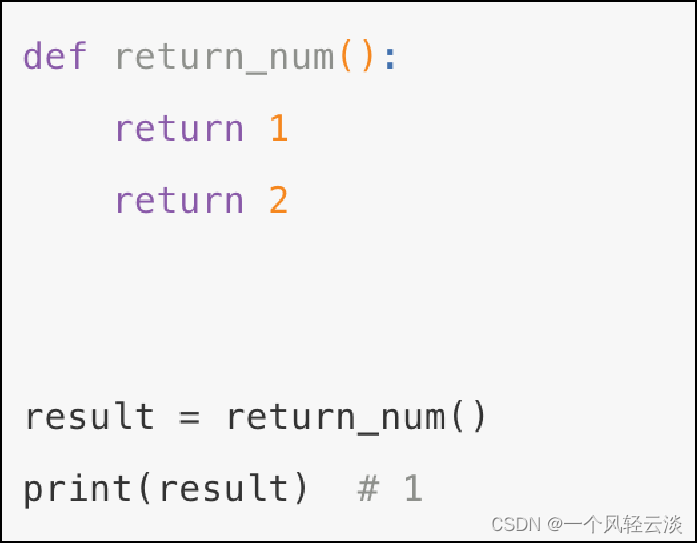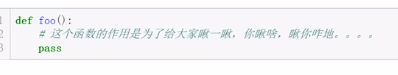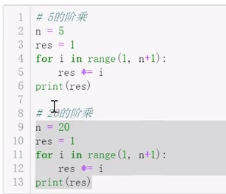# python函数

+关注继续查看

### 编程方法

面向对象               独门秘籍：类      class

### 函数定义

1、数学函数：
x是自变量，y是因变量；自变量的函数x的取值范围叫做这个函数的定义域；x的变化会引起y的变动。

2、函数式编程：

3、python中的函数定义方法：

    def test01(x):                             #def是定义函数的关键字；test是函数名；括号内是可定义形参；
"the function definitions"  #""里边是描述
x += 1                                  #泛指代码块或程序处理逻辑
return x                              #定义返回值

4、面向过程和函数式编程的区别之处

def test1():
'''test1'''
print("test1")
return 0             #有返回值

def test02():
'''test02'''
print("test02")             #没有返回值

test1()    #()代表调用test1函数；括号内可以有参数 也可以没有。
x=test1()
print(x)

test1
0

y=test02()
print(y)

test02
None

python中的面向过程，系统会默认返回一个None。

### 函数的三个特点

with open("test01","a") as f:
f.write('this is a stop')

def test1():
print("this is test1")
with open("test01", "a") as f:
f.write("stop stop")
def test02():
print("this is test02")
with open("test01", "a") as f:
f.write("stop stop")
def test3():
print("this is test3")
with open("test01", "a") as f:
f.write("stop stop")

test1()
test02()
test3()

def logger():
with open("test01","a") as f:
f.write('this is a stop\n')
def test1():
print("this is test1")
logger()
def test02():
print("this is test02")
logger()
def test3():
print("this is test3")
logger()

test1()
test02()
test3()

import time
time_format = "%Y-%m-%d %X"
time_now = time.strftime(time_format)
def logger():
with open("test01","a") as f:
f.write('\n%s this is a stop\n'%time_now)
def test1():
print("this is test1")
logger()
def test02():
print("this is test02")
logger()
def test3():
print("this is test3")
logger()

test1()
test02()
test3()

1.代码重用
2.保持一致性
3.可扩展性

### 函数返回值

def abc1():
print("aaaaaaa:")
return 0                  #return 结束逻辑；后边的代码不执行；
print("bbbbbbb:")
def abc2():
print("aaaaaaa:")
def abc3():
print("aaaaaaa:")
return 1,"hello",["a","b","c"],{"aaa":111}

x = abc1()                   #x接收的是abc函数的返回值0；
y = abc2()
z = abc3()
print(x)                     #return 结束逻辑；后边的代码不执行；
print(y)
print(z)

'''

aaaaaaa:
aaaaaaa:
aaaaaaa:
0
None
(1, 'hello', ['a', 'b', 'c'], {'aaa': 111})
'''

1.没有return返回值：返回None
2.return返回一个值：返回object （object就是你定义的返回值；python中的所有数据类型都是对象）
3.return返回多个值：返回tuple （元组形式返回）

### 函数参数

1、形参和实参

2、位置参数和关键字参数

def test01(x,y):          #x和y是形参
print(x)
print(y)

test01(1,2)              #1和2是实参;位置参数和形参要一一对应；
test01(x=1,y=2)      #关键参数与形参顺序无关；
#位置参数和关键数同时存在的时候，关键参数不能写在位置参数前面；

3、默认参数

def moren(x,y=6):       #y=6 是默认参数
print(x)
print(y)
moren(5)

5
6

4、参数组

def canshuzu(*args):        # *代表接收的参数是不固定的；*args把实参变成了一个元组；
print(args)
canshuzu(1,2,3,4,5)
'''

'''

def canshuzu2(x,*args):
print(x)
print(args)
canshuzu2(1,2,3,4,5)
'''

1
(2, 3, 4, 5)
'''

#*args：接收n个位置参数，转换成元组的形成
#**kwargs: 把n个关键字参数转换成字典方式

def canshuzu4(**kwargs):
print(kwargs)
print(kwargs['name'])
canshuzu4(name="aaa",age=111)

{'name': 'aaa', 'age': 111}
aaa
canshuzu4(**{"name":"aaa","age":222})

{'name': 'aaa', 'age': 222}
aaa

def canshuzu5(name,age=12,*args,**kwargs):
print(name)
print(age)
print(*args)
print(kwargs)
canshuzu5("aaa",age=22,job="it",)

aaa
22
#空的元组
{'job': 'it'}


### 函数局部变量和全局变量

namename = "AABBCC"                 #全局变量
def jubu(name):
namename = "aabbcc"               #局部变量
print("before change",name,namename)
name = "ABC"                    #这里的name是局部变量，这个函数就是这个变量的作用域；
print("after change",name)

name = "abc"                        #全局变量

jubu(name)                           #执行函数后的结果
print(name)                          #此处没有执行函数，直接调用变量
print(namename)                      #此处没有执行函数，直接调用变量
'''

before change abc aabbcc
after change ABC
abc
AABBCC
'''

局部变量：在子程序中（函数）定义的变量称为局部变量。
局部变量作用域是该变量的子程序；

全局变量作用域是整个程序；

当定义局部变量的子程序内，局部变量起作用；其他地方全局变量起作用。
字符串和整数 在局部变量情况下是不能修改全局变量的值；要想改变在局部变量前加global；
除了字符串和整数、元组以外高级点的类型（列表、字典、集合、类），局部变量可以改变全局变量的值；
 例子：设置局部变量修改全局变量
namename = "AABBCC"
def jubu(name):
global namename                 #设置局部变量修改全局变量
namename = "aabbcc"
print("before change",name,namename)
name = "ABC"                    #这里的name是局部变量，这个函数就是这个变量的作用域；
print("after change",name)
name = "abc"
jubu(name)
print(name)
print(namename)

before change abc aabbcc
after change ABC
abc
aabbcc

### 函数-递归

1.必须有一个明确的结束条件；
2.每次进入更深一层递归时，问题规模相比上次递归都应有所减少；
3.递归效率不高，递归层次过多会导致栈溢出（在计算机中，函数调用是通过栈（stack）这种数据结构实现的，每当进入一个函数调用，栈就会加一层栈帧，每当函数返回，栈就会减一层栈帧。由于栈的大小不是无限的，所以，递归调用的次数过多，会导致栈溢出。）
4.最大的递归层次是999层，超过 就报错；

def digui(n):
print(n)
return digui(n)
dict(0)                 #0是为了查看递归的次数,递归的次数最大为997次；然后就报错了

def digui2(n):
print(n)
if int(n/2) >0:
return digui2(int(n/2))
print(n)
digui2(10)

10
5
2
1
1

### 高阶函数

例如：
return z(x) + z(y)
print(result)

9.3

### 扩展：

本文转自506554897 51CTO博客，原文链接：http://blog.51cto.com/506554897/2070296，如需转载请自行联系原作者0 00 00 0python 函数深入

0 0python的函数

0 0Python：jpype模块调用Java函数
Python：jpype模块调用Java函数
0 0

Python语言基础 - 函数、面向对象、异常处理5452253

【科技少年】Python基础语法127424414101

Python语言基础 - 列表、元组、字典、集合5287320

Python 脚本进阶12814

Python语言基础 - 语法入门8936157

Python 脚本速查手册